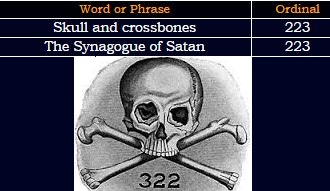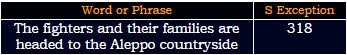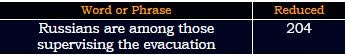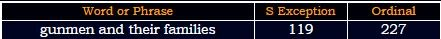CipherMar 19, 2017

Syrian city of Homs evacuation - 3/18/2017 - 314/318/227 coding

CNN's post - http://edition.cnn.com/2017/03/18/middleeast/syria-conflict-rebels-evacuated/index.html

I love catching smaller articles like this because the coding is always really easy to pick upIn Syrian city of Homs an orderly evacuation of rebels = 9+5 + 1+7+9+9+1+5 + 3+9+2+7 + 6+6 + 8+6+4+1 + 1+5 + 6+9+4+5+9+3+7 + 5+4+1+3+3+1+2+9+6+5 + 6+6 + 9+5+2+5+3+1 = 223 (Reduced)This story is coming on March 18th - written 3/18, and connecting to the first line of this story, the fact that story is coming on this date means that it will have circle and Saturn codingAlso we find 318 in story highlights318 is a number connecting to Pi
7/22 = 0.318
22/7 = 3.14

The state-run Syrian Arab News Agency is reporting that the first batch of "gunmen and their families" are leaving the city's Al-Waer neighborhood.Syrian Arab News Agency = 10+7+9+9+1+5 + 1+9+1+2 + 5+5+5+10 + 1+7+5+5+3+7 = 107 (S Exception)119+191+911 = 1221 - 12/21 is the first day of Capricorn (Ruled by Saturn) - Saturn = 119 (Francis Bacon)Star of David = 119 (Ordinal)All seeing eye = 119 (Ordinal)
227+272+722 = 1221
Russian supervision = 9+6+8+8+9+8+4 + 8+6+2+4+9+5+9+8+9+3+4 = 119 (Reverse Reduced)
"Evacuation" = 5+700+1+3+200+1+100+9+50+40 = 1109 (Jewish)
The Homs Media Center, an activist group, is reporting that 2,000 residents including 400 rebel fighters have left Al-Waer and are heading to Jarablus in the Aleppo countryside.
"Al-Waer" = 27+12+49+1+5+18 = 112 (Francis Bacon)
"Circle" = 24+18+9+24+15+22 = 112 (Reverse Ordinal)Also look at the coordinates - 36° N, 38° E - Syria = 8+2+9+9+8 = 36 (Reverse Reduced)
3/18/17 - 3+18+17 = 38

People are moving from "city of Homs" = 24+18+7+2 + 12+21 + 19+12+14+8 = 137 (Reverse Ordinal) - 137 is 33rd prime number
to "Jarabulus" = 1+1+9+1+2+3+3+3+10 = 33 (S Exception)
Also 2000 residents are heading to Jarabulus
"two thousand" = 7+4+12 + 7+19+12+6+8+26+13+23 = 137 (Reverse Ordinal)
Weird.. right? I think there's no reason to decode this story any further, it's clear why all these numbers appeared